Electron. J. Differential Equations, Vol. 2017 (2017), No. 206, pp. 1-20.

### Fractional elliptic systems with nonlinearities of arbitrary growth Edir Junior Ferreira Leite

Abstract:
In this article we discuss the existence, uniqueness and regularity of solutions of the following system of coupled semilinear Poisson equations on a smooth bounded domain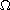in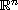: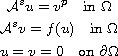where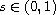and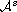denote spectral fractional Laplace operators. We assume that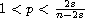, and the function f is superlinear and with no growth restriction (for example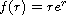); thus the system has a nontrivial solution. Another important example is given by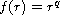. In this case, we prove that such a system admits at least one positive solution for a certain set of the couple (p,q) below the critical hyperbola

Submitted June 10, 2017. Published September 7, 2017.
Math Subject Classifications: 35J65, 49K20, 35J40.
Key Words: Fractional elliptic systems; critical growth; critical hyperbola.

Show me the PDF file (320 KB), TEX file for this article.Edir Junior Ferreira Leite Departamento de Matemática Universidade Federal de Viçosa CCE, 36570-000, Viçosa, MG, Brazil email: edirjrleite@ufv.br